# Excel Applications for Accounting Principles

## Quiz 28 :Statistics and Linear Regression StatsLooking for Accounting Homework Help?

## Quiz 28 :Statistics and Linear Regression StatsPROBLEM DATA A full year analysis of maintenance costs at Riverglen Manufacturing is as follows: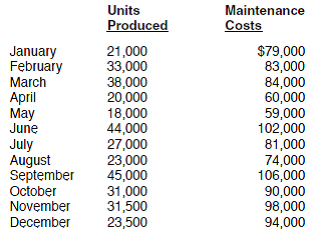REQUIREMENT The controller of Riverglen has asked you to calculate some statistics on this data. She is also interested in estimating the fixed and variable elements of the maintenance costs using linear regression. Review the worksheet STATS that follows these requirements.
Free
Essay

Open and review the worksheet indicated. In this problem you will calculate statistics on the data given for a company. You will use several functions in the worksheet to calculate this data. The unedited file will look like this: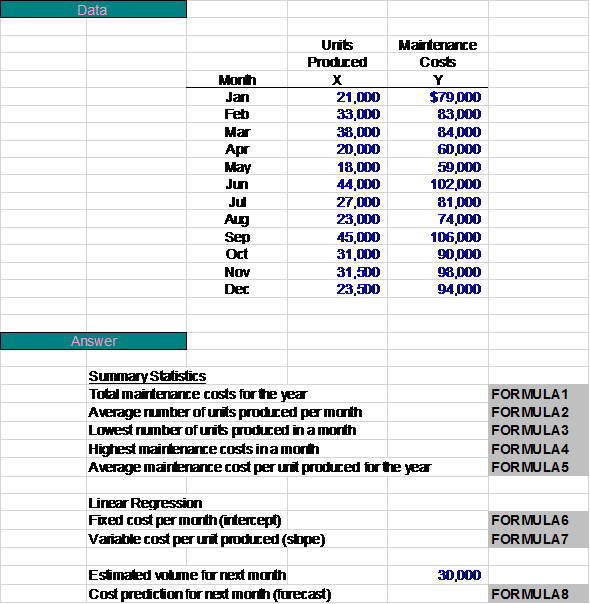PROBLEM DATA A full year analysis of maintenance costs at Riverglen Manufacturing is as follows:REQUIREMENT Open the file STATS from the website for this book at cengagebrain.com. Enter the formulas where indicated on the worksheet. January is the next month and Riverglen is predicting 30,000 units produced. Hint: Most of formulas are statistical functions in Excel. See Appendix A of Excel Quick for a discussion of these functions. Enter your name in cell A1. Print the worksheet when done. Also print your formulas. Save your completed file as STATS2. Check figure: Cost prediction for January (cell F38), \$84,713.
Free
Essay

Open the file listed. Enter the formulas where indicated on the worksheet using cell references so that if any data is modified, the figures will automatically update. Most of the formulas can be found in the statistics section. Be sure to include any additional data in the problem. When you have entered your formulas, your worksheet should look like this: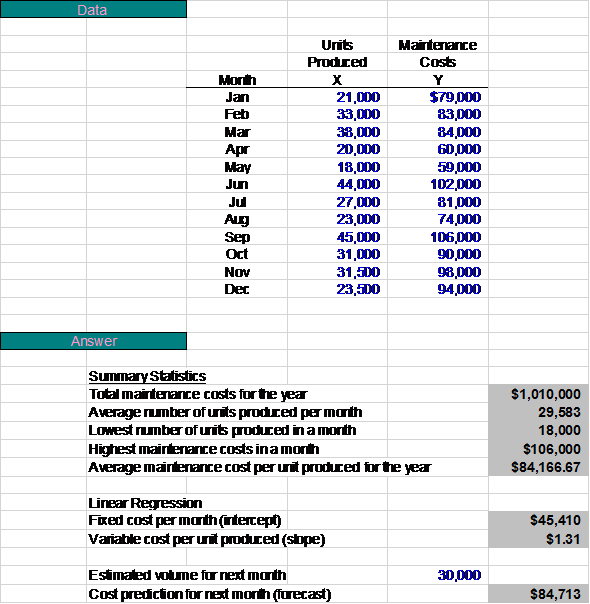WHAT-IF ANALYSIS Use the worksheet to complete the following table of other possible outcomes for January: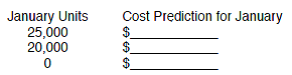Does your answer at zero units of production look familiar It should. Why
Free
Essay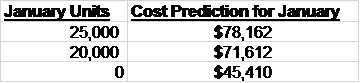The cost prediction for January at zero estimated units is the same amount as the fixed cost per month, which is the intercept.